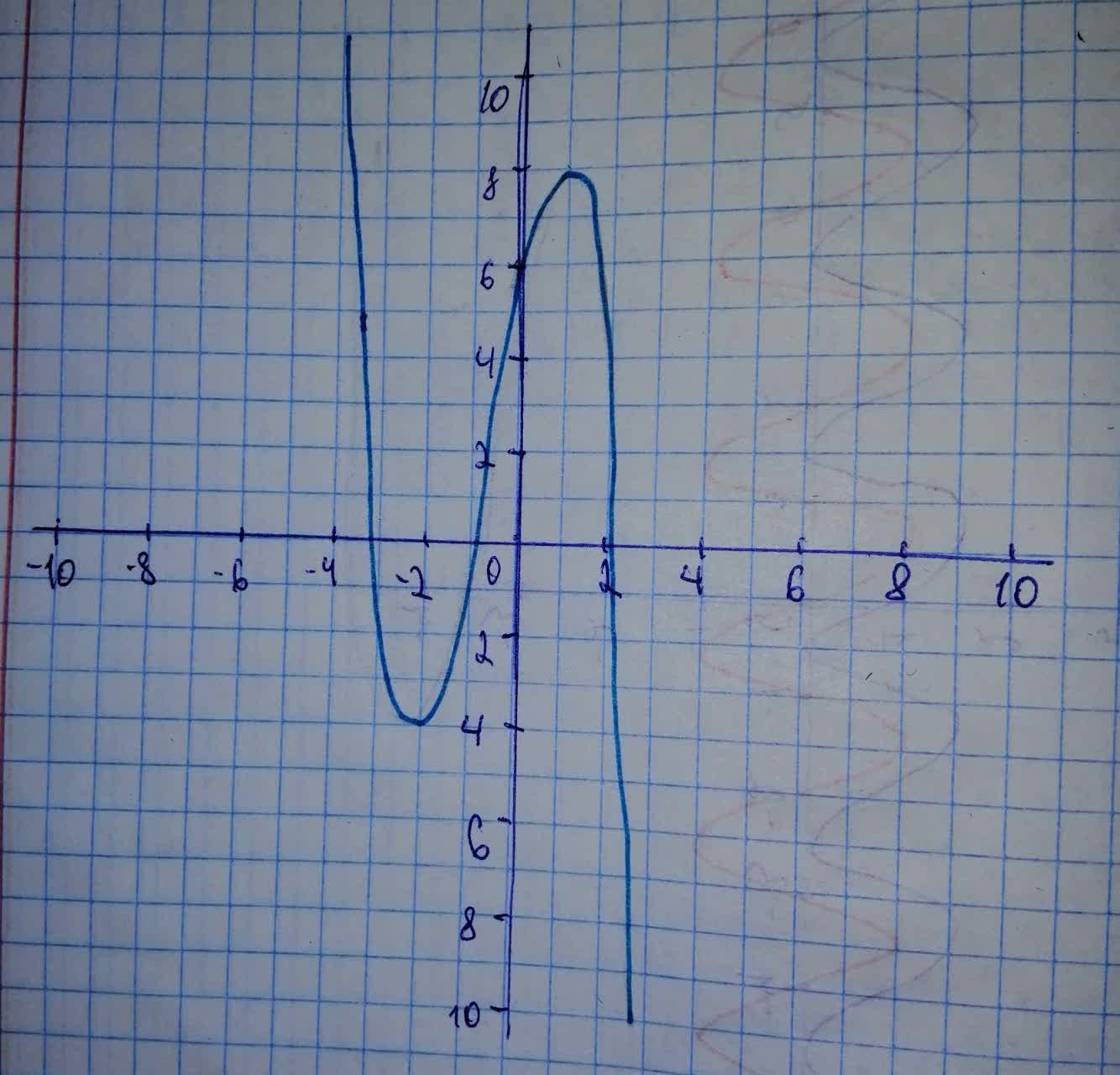# Polynomial function graphs have similarities depending on their degree. Explain how you can determine the best regression model by using finite differences. Then determine the end behavior of the graph based on the degree of a function and information gathered from a data table.rocedwrp 2020-11-08 Answered
Polynomial function graphs have similarities depending on their degree. Explain how you can determine the best regression model by using finite differences. Then determine the end behavior of the graph based on the degree of a function and information gathered from a data table.
You can still ask an expert for help

## Want to know more about Exponential growth and decay?

• Live experts 24/7
• Questions are typically answered in as fast as 30 minutes
• Personalized clear answers

Solve your problem for the price of one coffee

• Math expert for every subject
• Pay only if we can solve itBrittany Patton

Step 1
Determining the best regression model by using finite differences. The method of finite differences is used for curve fitting with polynomial models. It is best introduced by an example as shown in table given below. For the sequence of x value inputs at the regularly spaced points the y value outputs are shown in the table.
Table 1 is given below.
From the table, we can understand that this is third difference finite function of third degree.
This explains
$f\left(x\right)=a{x}^{3}+b{x}^{2}+cx+d.$
Step 2
Here we need to find the value of a, b, c and d
$a=\frac{-6}{3!}$
$a=\frac{-6}{6}$
$a=-1$
Step 3
Taking $x=0,-1,1$ for calculating the value of rest of the co-efficients.
$f\left(x\right)=-{x}^{3}+b{x}^{2}+cx+d$
$f\left(-1\right)=1+b-c+6$
$x=-1$
$y=0$
$0=b-c+7$
$-7=\left(b-c\right)$
$\begin{array}{|ccccc|}\hline x& y& \text{First Difference}& \text{Second Difference}& \text{Third Difference}\\ -3& 0& \text{EMPTY VALUE}& \text{EMPTY VALUE}& \text{EMPTY VALUE}\\ -2& -4& -4-0=-4& \text{EMPTY VALUE}& \text{EMPTY VALUE}\\ -1& 0& 0-\left(-4\right)=4& 4-\left(-4\right)=8& \text{EMPTY VALUE}\\ 0& 6& 6-0=6& 2& 2-8=-6\\ 1& 8& 8-6=2& -4& -6\\ 2& 0& 0-8=-8& -10& -6\\ 3& -24& -24-0=24& -16& -6\\ \hline\end{array}$
Also

$8=b+c+5$
$b+c=3$
Moreover,
$f\left(0\right)=-0+0+0+d$
$x=0$
$y=6$
$6=d$
Thus, finding b and c by subtracting and adding equation (1) and (2)
$2b=-4$
$b=-2$
$2c=10$
$c=5$
At last the finite function becomes:
$f\left(x\right)=-{x}^{3}-2{x}^{2}+5x+6$
Step 4
Sketching a grph from the function found above
Thus, end behaviour of $f\left(x\right)=-{x}^{3}-2{x}^{2}+5x+6$
is described from the table as below
As and AsWe have step-by-step solutions for your answer!